Question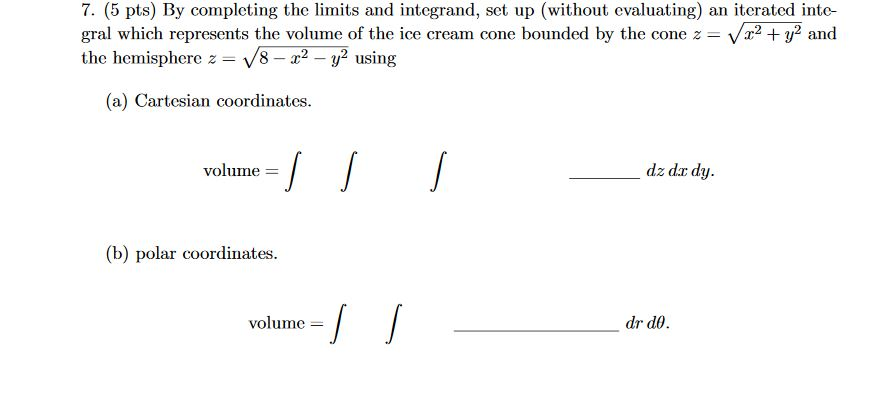7. (5 pts) By completing the limits and integrand, set up (without evaluating) an iterated inte-gral which represents the volume of the ice cream cone bounded by the cone z=√x2+y2andthe hemisphere z=√8−x2−y2using(a) Cartesian coordinates.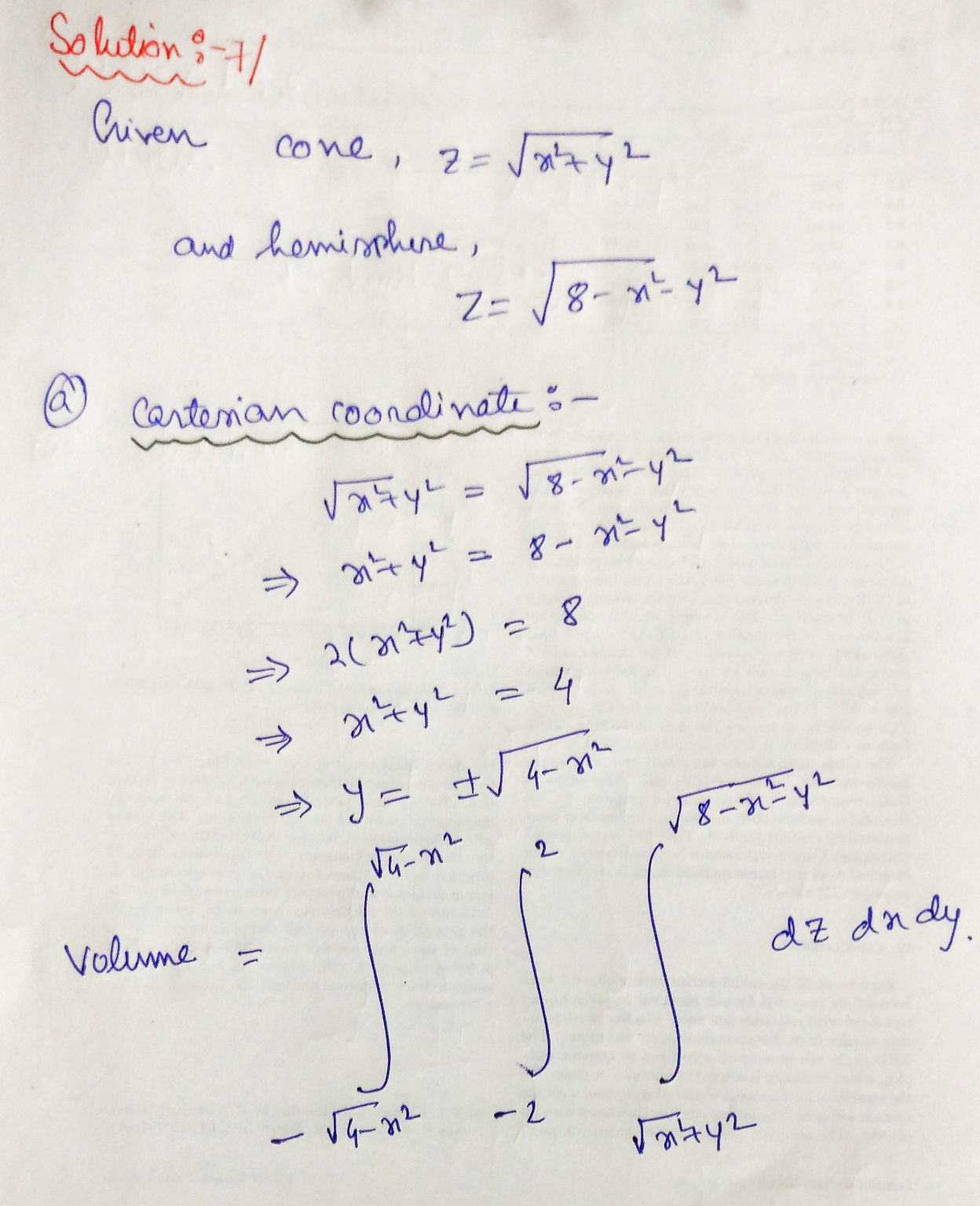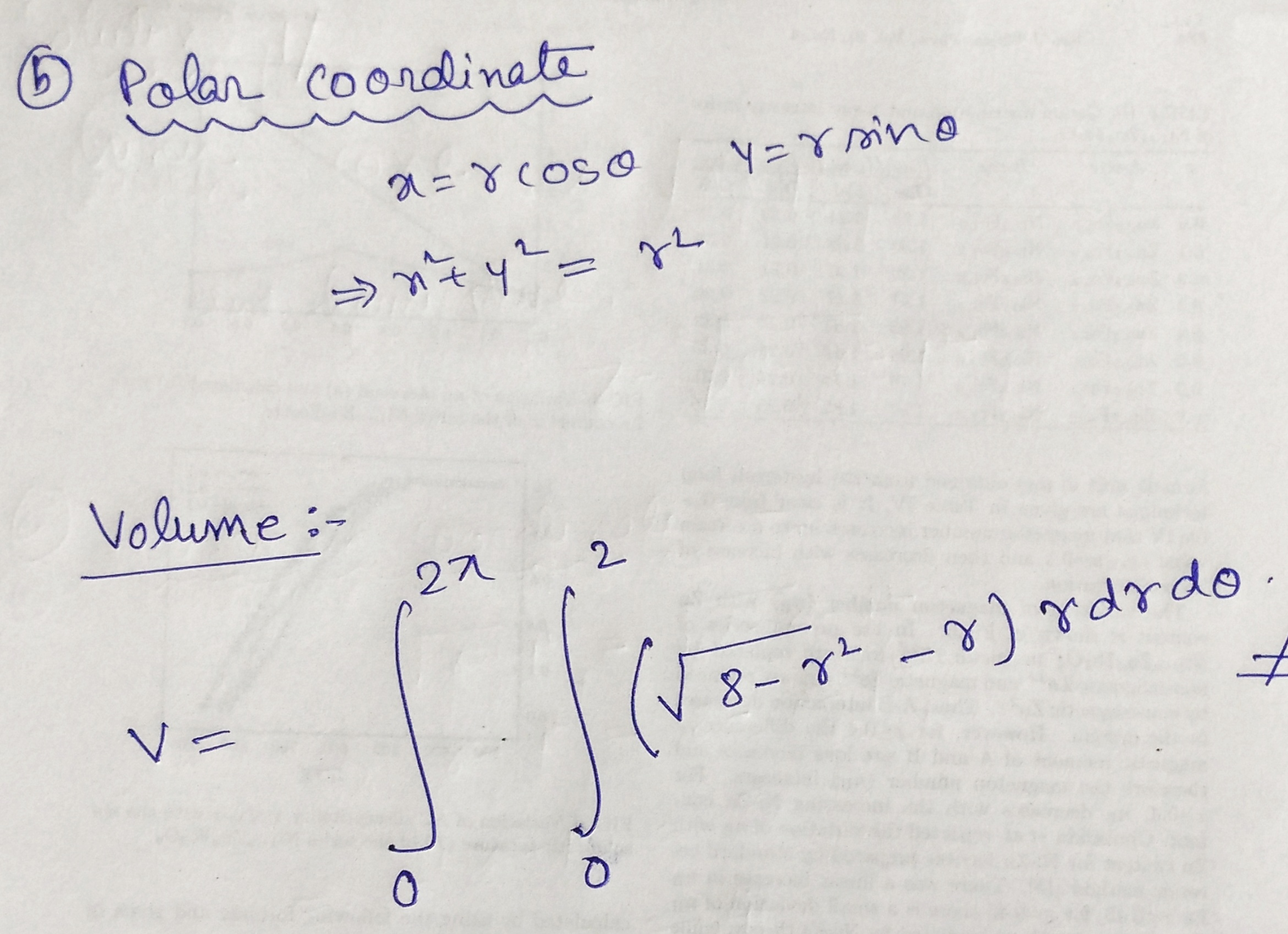#### Earn Coins

Coins can be redeemed for fabulous gifts.

Similar Homework Help Questions
• ### 7. (5 pts) By completing the limits and integrand, set up (without evaluating) an iterated inte-...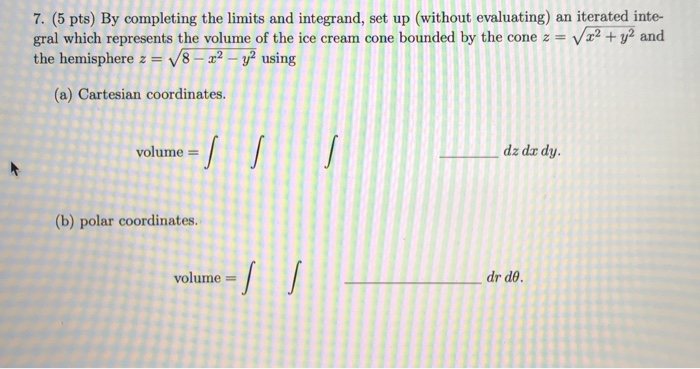7. (5 pts) By completing the limits and integrand, set up (without evaluating) an iterated inte- gral which represents the volume of the ice cream cone bounded by the cone z = V x2 + y2 and the hemisphere z = V8 - 22 - y2 using (a) Cartesian coordinates. volume = dz dar dy. (b) polar coordinates. volume = dr de.

• ### 7. (5 pts) By completing the limits and integrand, set up (without evaluating) an iterated inte-...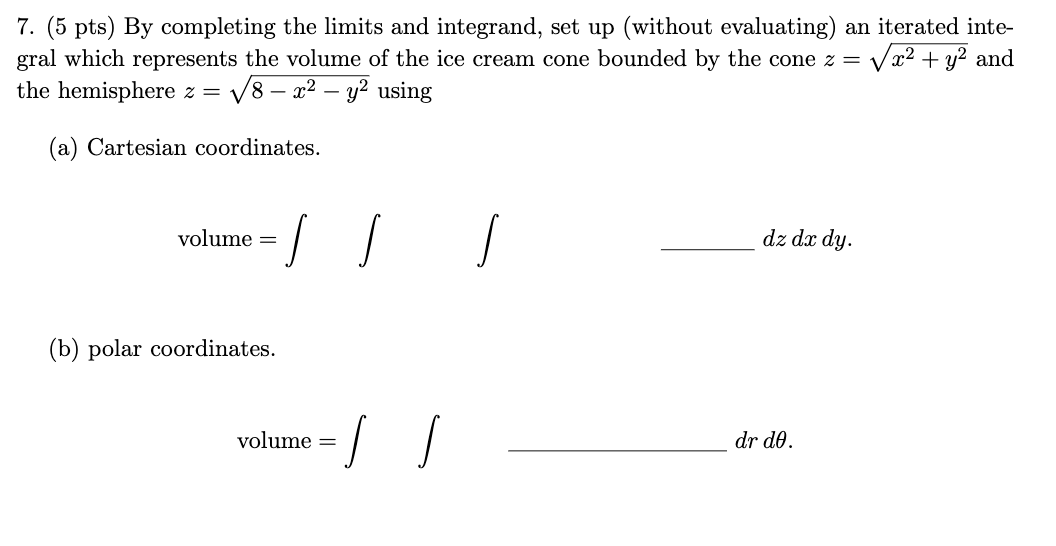7. (5 pts) By completing the limits and integrand, set up (without evaluating) an iterated inte- gral which represents the volume of the ice cream cone bounded by the cone z = V x2 + y2 and the hemisphere z = V8 – x2 - y2 using (a) Cartesian coordinates. volume = dz dx dy. (b) polar coordinates. volume = I dr de.

• ### 6. (4 pts) Consider the double integral∫R(x2+y)dA=∫10∫y−y(x2+y)dxdy+∫√21∫√2−y2−√2−y2(x2+y)dxdy.(a) Sketch the region of integration R in Figure 3.(b)...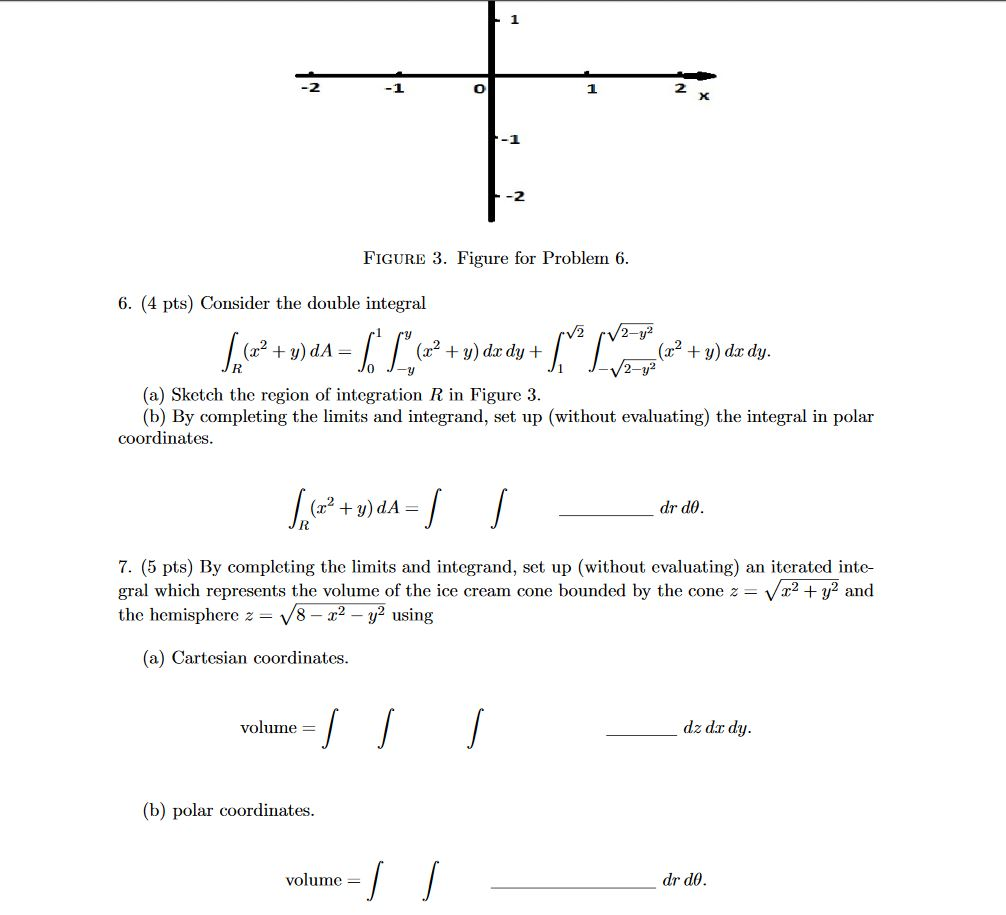6. (4 pts) Consider the double integral∫R(x2+y)dA=∫10∫y−y(x2+y)dxdy+∫√21∫√2−y2−√2−y2(x2+y)dxdy.(a) Sketch the region of integration R in Figure 3.(b) By completing the limits and integrand, set up (without evaluating) the integral in polar coordinates.∫R(x2+y)dA=∫∫drdθ.7. (5 pts) By completing the limits and integrand, set up (without evaluating) an iterated inte-gral which represents the volume of the ice cream cone bounded by the cone z=√x2+y2andthe hemisphere z=√8−x2−y2using(a) Cartesian coordinates.volume =∫∫∫dz dxdy.(b) polar coordinates.volume =∫∫drdθ. -1 -2 FIGURE 3. Figure for Problem 6. 6. (4 pts)...

• ### 6. (4 pts) Consider the double integral∫R(x2+y)dA=∫10∫y−y(x2+y)dxdy+∫√21∫√2−y2−√2−y2(x2+y)dxdy.(a) Sketch the region of integrationRin Figure 3.(b) By completing...6. (4 pts) Consider the double integral∫R(x2+y)dA=∫10∫y−y(x2+y)dxdy+∫√21∫√2−y2−√2−y2(x2+y)dxdy.(a) Sketch the region of integrationRin Figure 3.(b) By completing the limits and integrand, set up (without evaluating) the integral in polar coordinates. -1 -2 FIGURE 3. Figure for Problem 6. 6. (4 pts) Consider the double integral V2 /2-y² + = (x2 + y) dx dy + + y) do dy. 2-y2 (a) Sketch the region of integration R in Figure 3. (b) By completing the limits and integrand, set up (without evaluating)...

• ### Can anyone help me figure out the limits and what the function would be? Thank you!...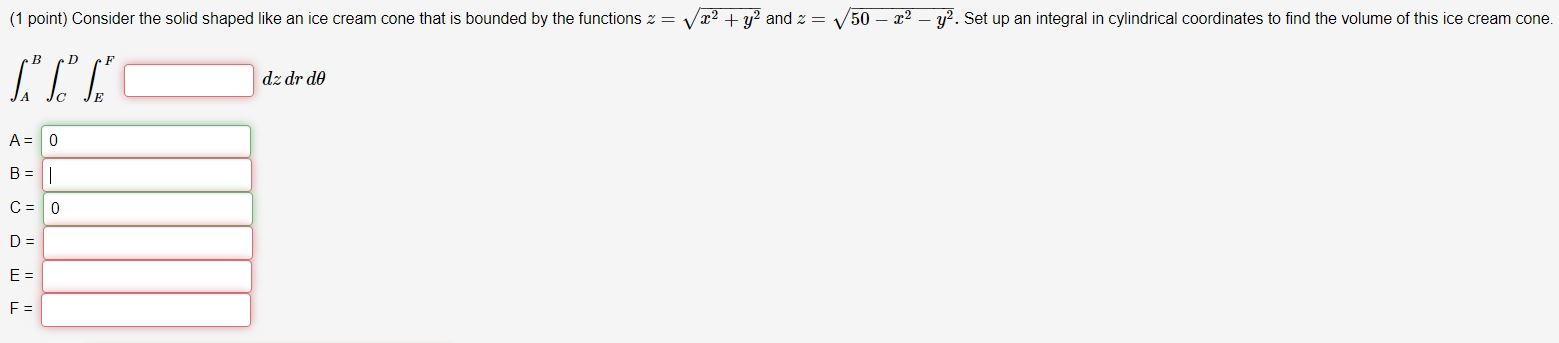Can anyone help me figure out the limits and what the function would be? Thank you! (1 point) Consider the solid shaped like an ice cream cone that is bounded by the functions 2= v x2 + y2 and 2 = 50 - 22 - y2. Set up an integral in cylindrical coordinates to find the volume of this ice cream cone. B D F "T" dz dr de A = 0 B = - C = 0 D =...

• ### please write neatly and no script! 8. (10 points) (a) Using rectangular coordinates, set up an...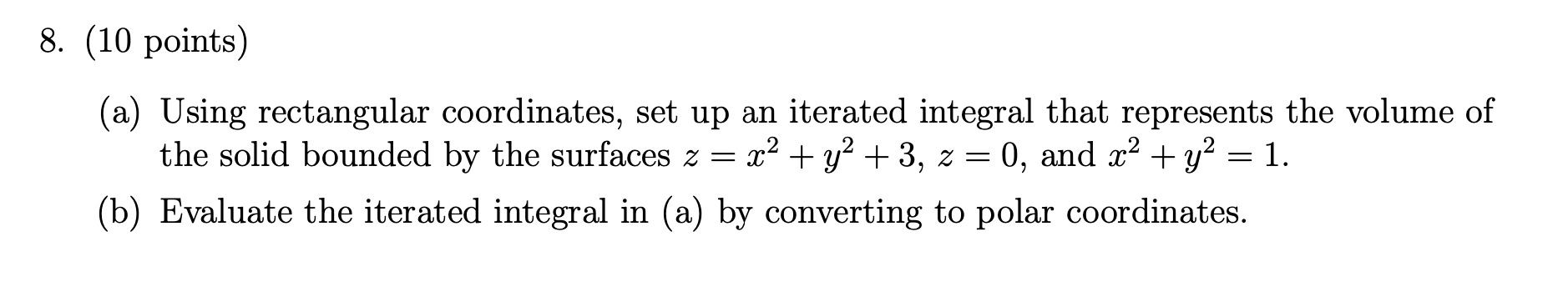please write neatly and no script! 8. (10 points) (a) Using rectangular coordinates, set up an iterated integral that represents the volume of the solid bounded by the surfaces z = x2 + y2 +3, z = 0, and x2 + y2 = 1. (b) Evaluate the iterated integral in (a) by converting to polar coordinates.

• ### 6. (4 pts) Consider the double integral∫R(x2+y)dA=∫10∫y−y(x2+y)dxdy+∫√21∫√2−y2−√2−y2(x2+y)dxdy.(a) Sketch the region of integration R in Figure 3.(b)...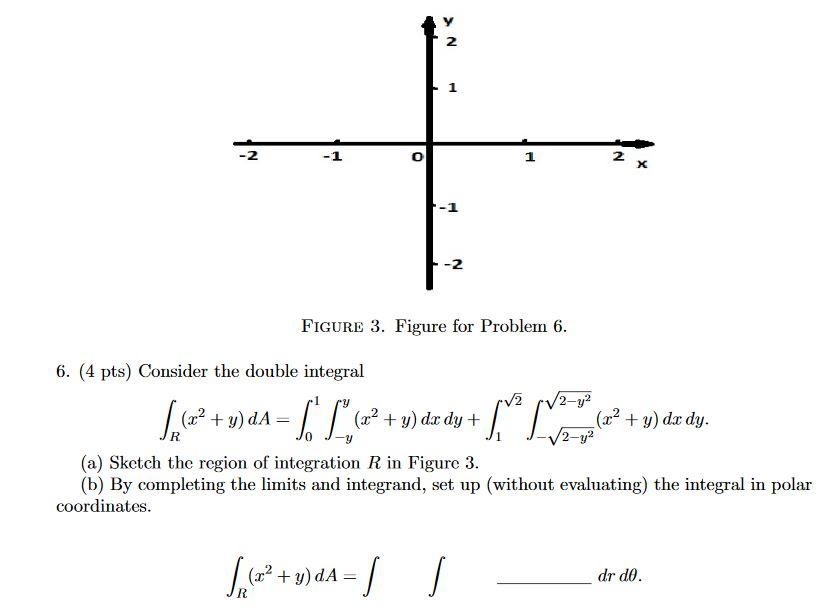6. (4 pts) Consider the double integral∫R(x2+y)dA=∫10∫y−y(x2+y)dxdy+∫√21∫√2−y2−√2−y2(x2+y)dxdy.(a) Sketch the region of integration R in Figure 3.(b) By completing the limits and integrand, set up (without evaluating) the integral in polar coordinates. 2 1 2 X -2 FIGURE 3. Figure for Problem 6. 6. (4 pts) Consider the double integral V2 2-y2 (2? + y) dA= (32 + y) dx dy + (x2 + y) dx dy. 2-y? (a) ketch the region of integration R in Figure 3. (b) By completing...

• ### Question Use cylindrical coordinates to set up the triple integral needed to find the volume of...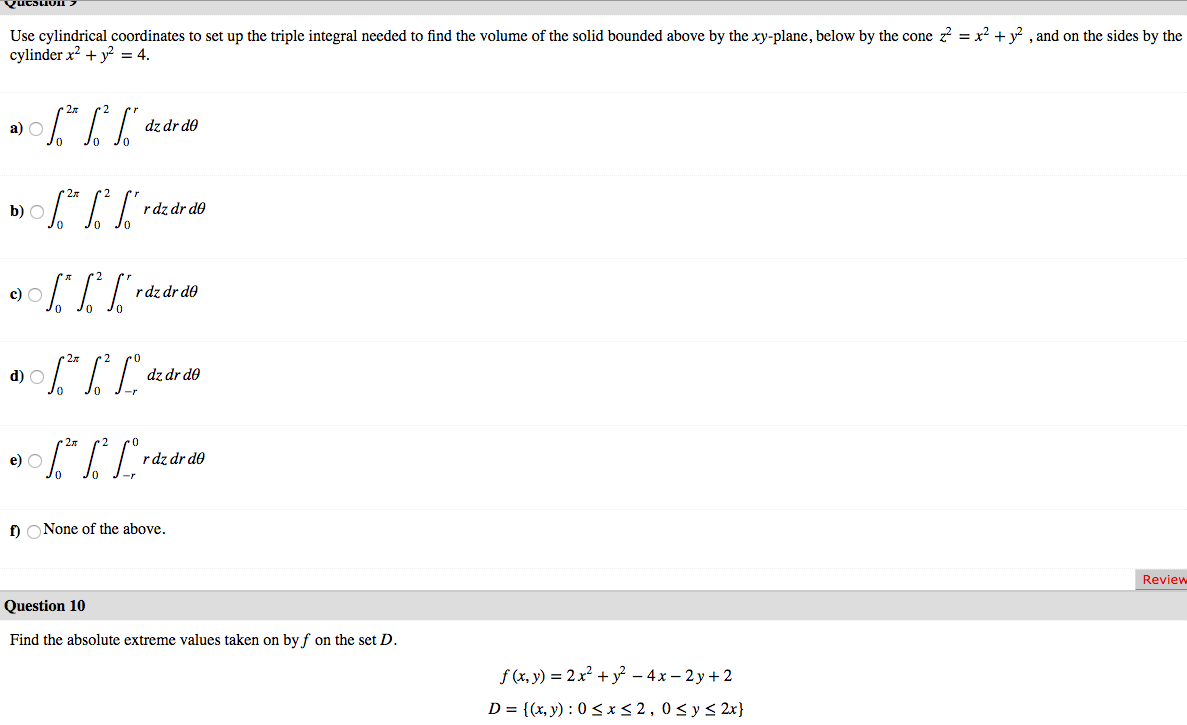Question Use cylindrical coordinates to set up the triple integral needed to find the volume of the solid bounded above by the xy-plane, below by the cone z = x2 + y2 , and on the sides by the cylinder x2 + y2 = 4. a) 06.* %* ["dz dr do b) \$* * S*rde de do JO 0% ] raz dr do a) \$** [Lºdz dr do 0906.*|*Lºrdz dr do 2 po dz dr do Jo J- O J-...

• ### solutions are labeled a to c at the bottom. can you explain what the r stands for. I'm assuming x2 + y2 Write iterated integrals for each of the given caleu- Question 7 (5 pts each] lations. D...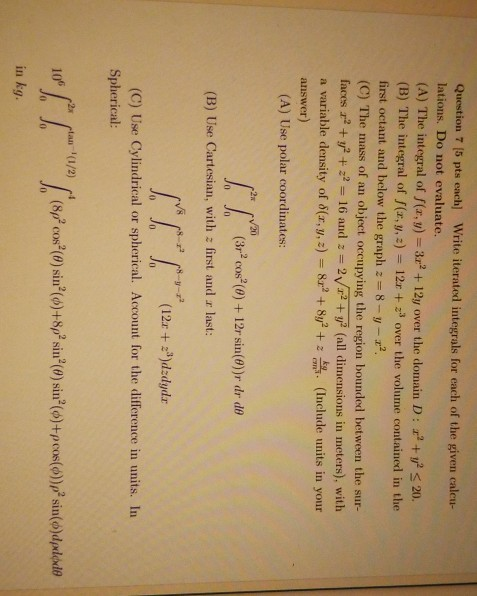solutions are labeled a to c at the bottom. can you explain what the r stands for. I'm assuming x2 + y2 Write iterated integrals for each of the given caleu- Question 7 (5 pts each] lations. Do not evaluate. (A) The integral of f(x,y) 32 + 12y over the domain D: +20 (B) The integral of f(x, y,) first octant and below the graph z 8-y 2 (C) The mass of an object occupying the region bounded between the...

• ### 1) a.(20 pts) Set up the integral corresponding to the volume of the solid bounded above by the sphere x2+y2 + z2 16 and below by the cone z2 -3x2 + 3y2 and x 2 0 and y 20. You may want to graph...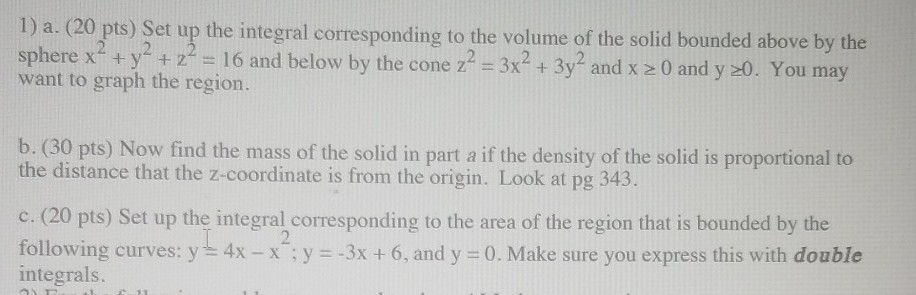1) a.(20 pts) Set up the integral corresponding to the volume of the solid bounded above by the sphere x2+y2 + z2 16 and below by the cone z2 -3x2 + 3y2 and x 2 0 and y 20. You may want to graph the region. b. (30 pts) Now find the mass of the solid in part a if the density of the solid is proportional to the distance that the z-coordinate is from the origin. Look at pg...